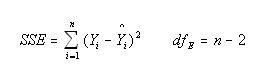# Residual Sum of Squares

Posted in Statistics, Total Reads: 1562

## Definition: Residual Sum of Squares

Residual Sum of Squares is a statistical technique which is used to measure the amount of variance in a set of data points that cannot be explained by a regression model.

Residual sum of squares is calculated by subtracting the predicted estimated values from the real values available, which is then squared and added.Where Yi is the ith value of the variable to be predicted and Yi hat is the predicted value of Y in a standard linear regression model where yi=a+bxi+c

Browse the definition and meaning of more terms similar to Residual Sum of Squares. The Management Dictionary covers over 7000 business concepts from 6 categories. This definition and concept has been researched & authored by our Business Concepts Team members.

Search & Explore : Management Dictionary

Similar Definitions from same Category: